Author: Oscar Cronquist Article last updated on March 10, 2022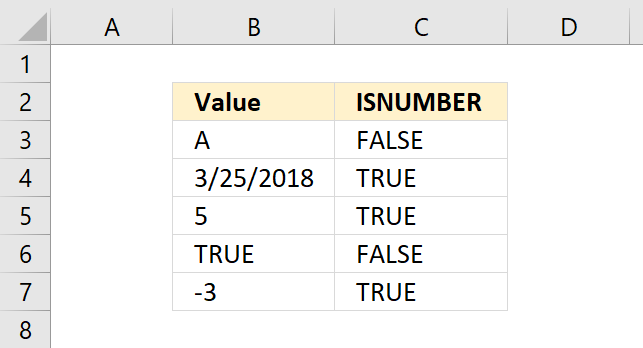The ISNUMBER function checks if a value is a number, returns TRUE or FALSE.

ISNUMBER(value)

## 2. ISNUMBER Function Arguments

 value Required. The value you want to check if it is a number.

## 3. ISNUMBER function exampleThe image above shows the ISNUMBER function in column C. The first value is text value "A" in cell B3, the ISNUMBER function returns FALSE meaning the value is not a number.

The second value in cell B4 contains an Excel date, it contains a number formatted as date. The ISNUMBER function returns TRUE.

The third value is number 5 and the ISNUMBER function returns TRUE, this also applies to negative numbers and 0 (zero).

The fourth value is a boolean value, the ISNUMBER function returns FALSE. Boolean values TRUE and FALSE are not numbers.

Formula in cell D3:

=ISNUMBER(B3)

## 4. ISNUMBER function not working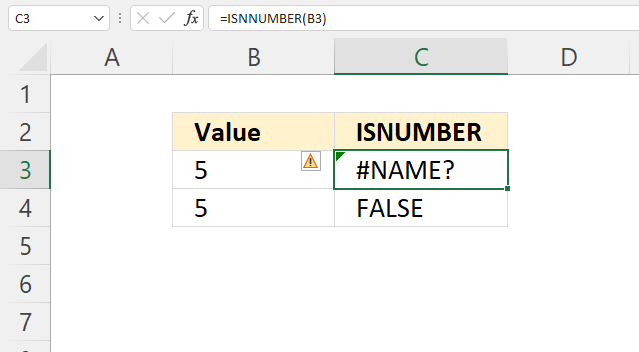Check your spelling, a #NAME? error is shown if a function name is unrecognized.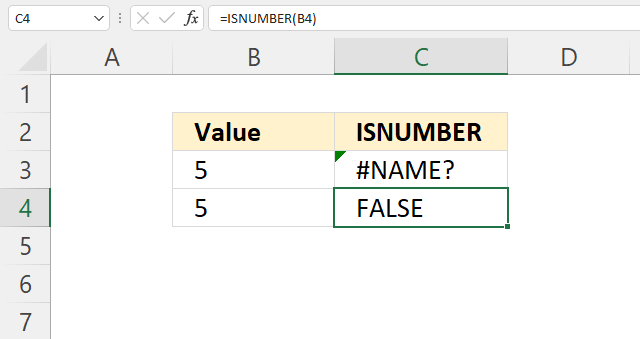The image above shows the ISNUMBER function returning the boolean value FALSE in cell C4 despite the fact that cell B4 contains a number.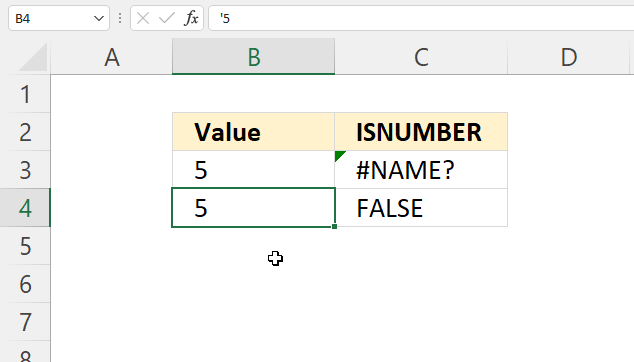We can see that cell B4 contains an apostrophe before the actual number. This makes Excel identify the number as a text value, however, the apostrophe is not shown in the cell making it very hard to spot.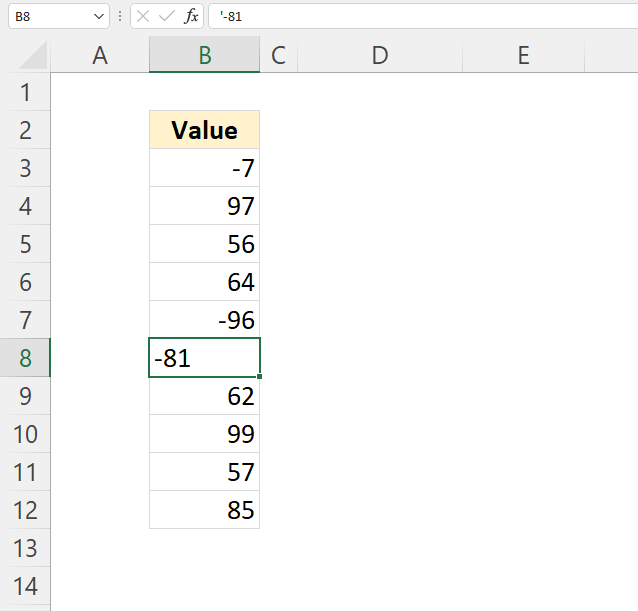Excel defaults to right-aligned values for numbers and left-aligned values for text values, see the image above. This is not displayed if you use custom cell formatting.

## 5. Identify numbers stored as text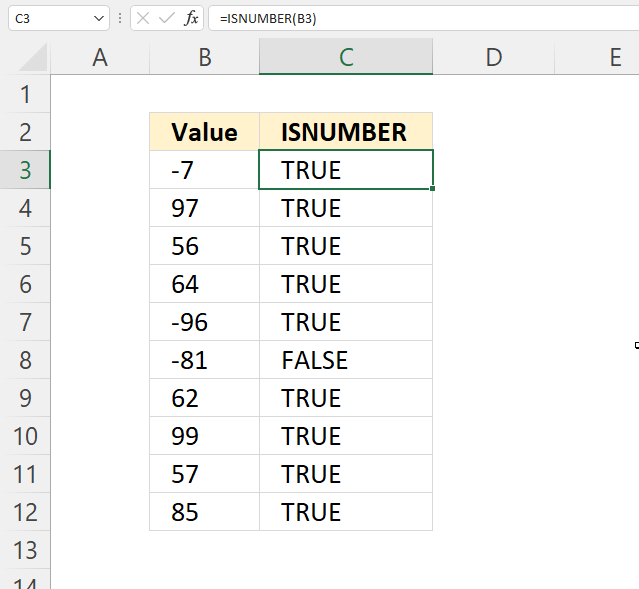The image above demonstrates how to identify numbers stored as text in a column, the ISNUMBER function returns FALSE if a value is not a number.

Cell C8 contains boolean value FALSE, the corresponding value in cell B8 contains '-81 which makes Excel think this value is a text value. This may happen if you import data from external sources like databases or web pages.

Formula in cell C3:

=ISNUMBER(B3)

It is not necessary to use a formula for each value, you can use a single array formula to process all values in cell range B3:B12. Read the next section to find out how.

### 5.1 Identify numbers stored as text in a cell range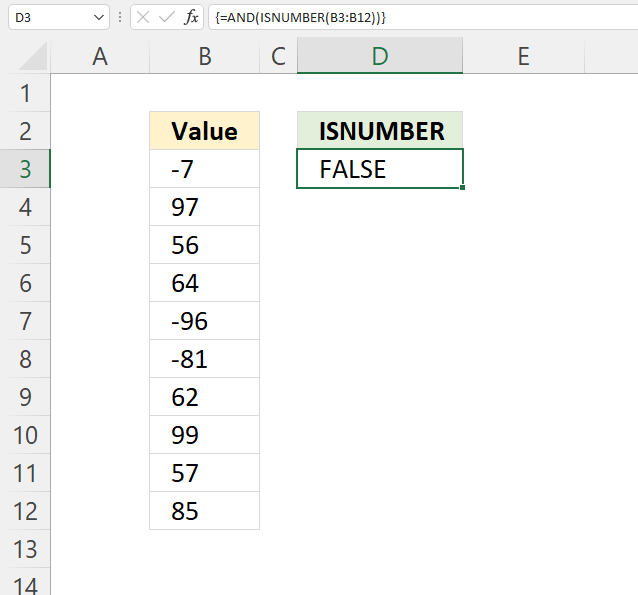The array formula in cell D3 checks if the values in cell range B3:B12 are all numbers. It looks like they all are numbers, however, the ISNUMBER function has identified at least one cell value containing a text value.

The formula returns TRUE if all values are numbers and FALSE if at least one value is not a number.

Array formula in cell D3:

=AND(ISNUMBER(B3:B12))

#### 5.1.1 How to enter an array formula

Excel 365 users can skip the below steps, enter the formula as a regular formula.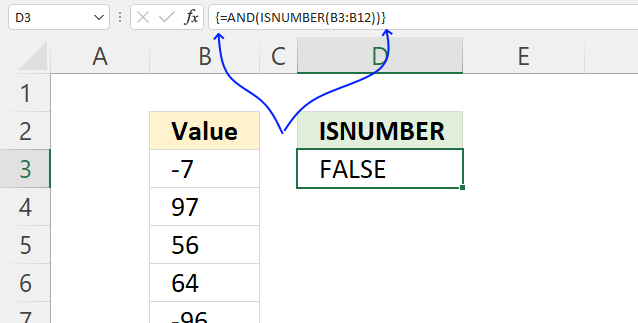1. Doublepress with left mouse button on cell D3 with the left mouse button.
2. Enter the above array formula.
3. Press and hold CTRL + SHIFT simultaneously.
4. Press ENTER once.
5. Release all keys.

The array formula begins and ends with curly brackets, see the image above. They appear automatically, don't enter these characters yourself.

#### 5.1.2 Explaining formula in cell D3

Step 1 - Check if the value is a number

ISNUMBER(B3:B12)

becomes

ISNUMBER({-7; 97; 56; 64; -96; "-81"; 62; 99; 57; 85})

and returns

{TRUE; TRUE; TRUE; TRUE; TRUE; FALSE; TRUE; TRUE; TRUE; TRUE}.

Step 2 - Check if all boolean values are TRUE

The AND function returns TRUE if all values are TRUE.

AND(logical1, [logical2], ...)

AND(ISNUMBER(B3:B12))

becomes

AND({TRUE; TRUE; TRUE; TRUE; TRUE; FALSE; TRUE; TRUE; TRUE; TRUE})

and returns FALSE. All values are not TRUE.

## 6. Highlight numbers stored as text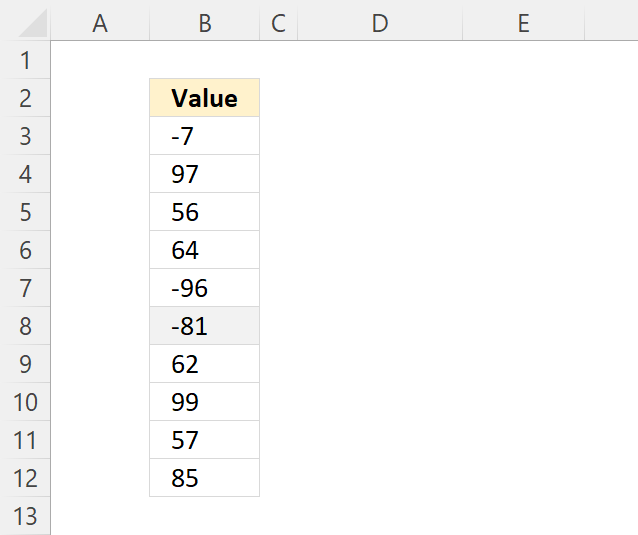The image above shows numbers in cell range B3:B12, one cell is highlighted light-grey so you can easily spot non-numbers. Here is how you can do the same:

1. Select cell range B3:B12.
2. Go to tab "Home" on the ribbon.
3. Press with mouse on the "Conditional Formatting" button on the ribbon. A popup menu appears.
4. Press with mouse on "New Rule...".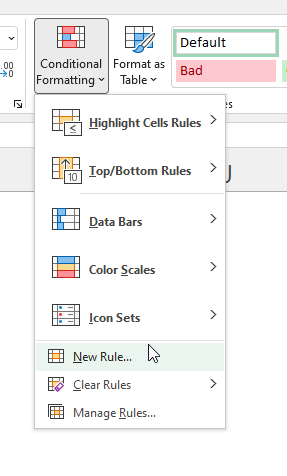5. A dialog box appears. Press with mouse on "Use a formula to determine which cells to format".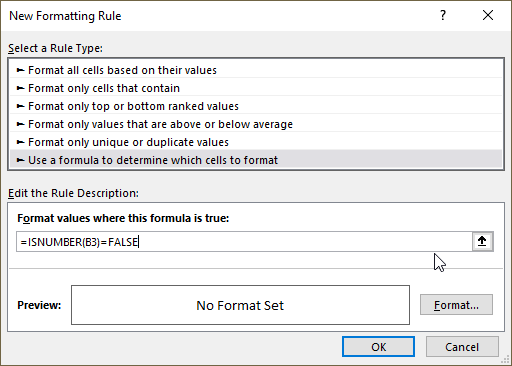6. Type the following formula:
=ISNUMBER(B3)=FALSE
7. Press with left mouse button on "Format..." button. Another dialog box appears.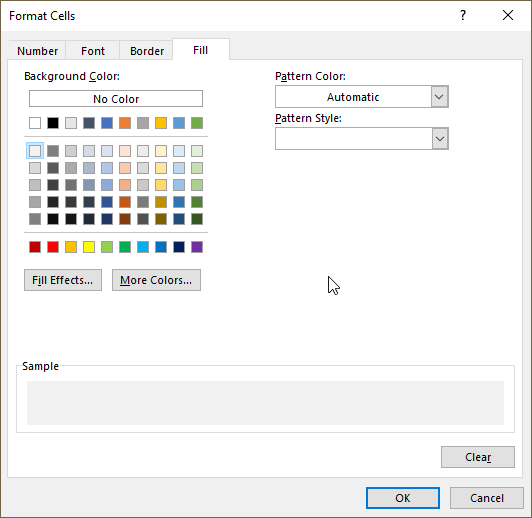8. Press with left mouse button on tab "Fill".
9. Pick a color to highlight cells with.
10. Press with left mouse button on OK button.
11. Press with left mouse button on OK button.

### 6.1 Sort highlighted numbers stored as text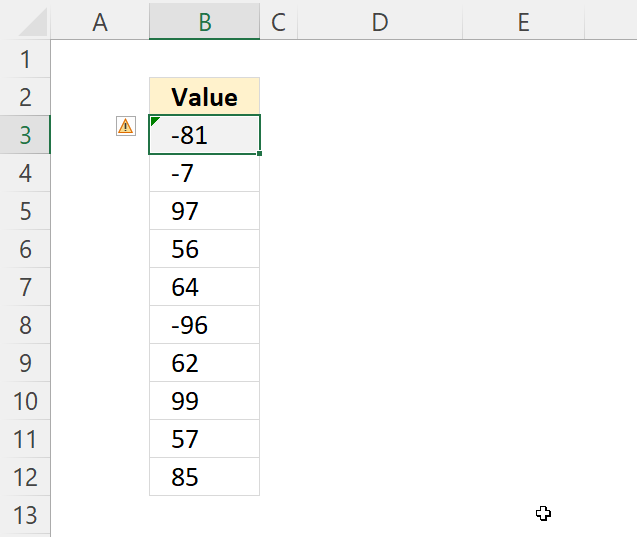You can sort cells based on cell color to quickly find numbers stored as text in larger data sets. Here is how:

1. Select the data range with the mouse.
2. Press with right mouse button on on the selected cell range. A popup menu appears.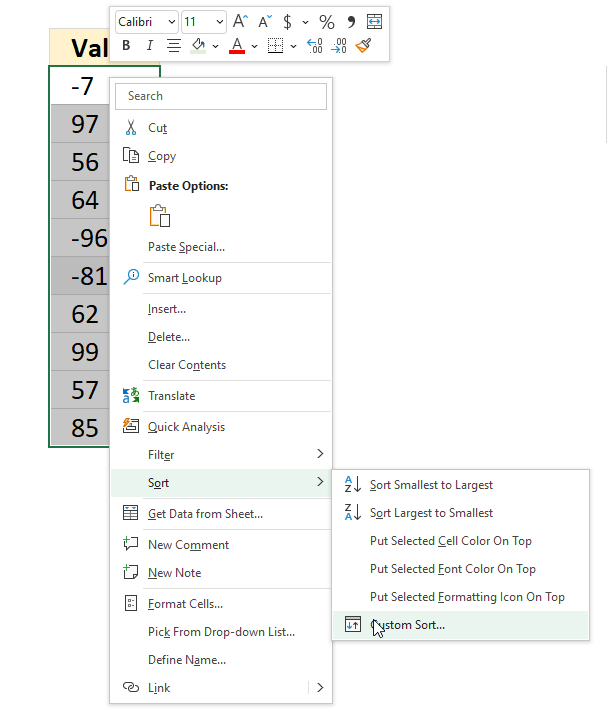3. Press with mouse on "Sort".
4. Press with mouse on "Custom Sort...". A dialog box appears.
5. Sort on "Cell Color".
6. Pick the color below "Order", see the image below.
7. Select "On Top".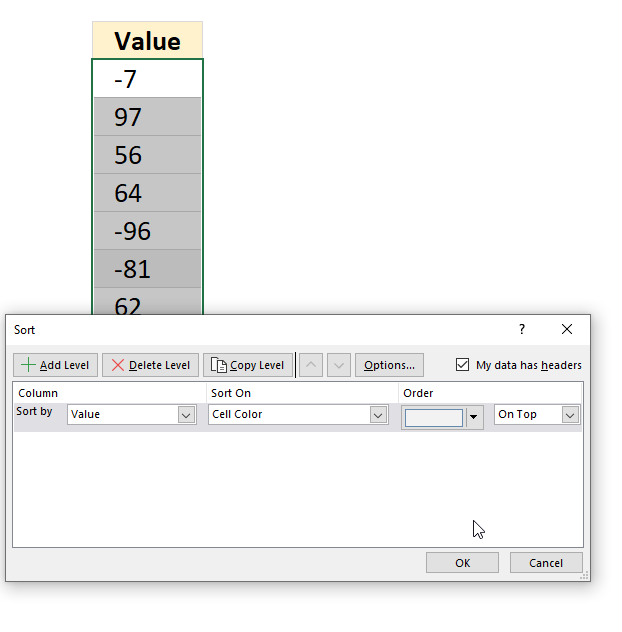8. Press with left mouse button on OK.

## 7. Can the ISNUMBER function handle error values?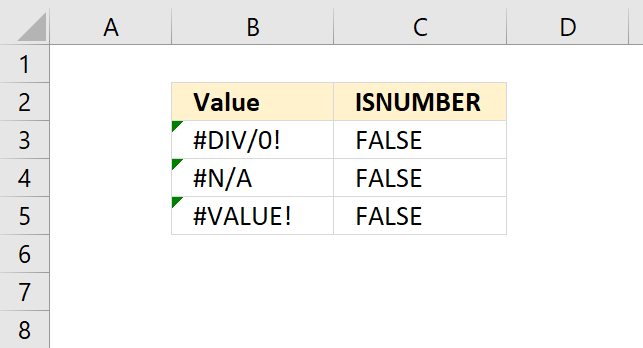The ISNUMBER function is really useful sometimes because it returns FALSE also from error values, as well. Most Excel functions return an error if they are fed with an error value, however, not all of them.

Most Excel functions that begin with IS handle error values. The image above shows three different error values, #DIV/0, #N/A, and #VALUE! errors. The ISNUMBER function returns FALSE for those values.

## 8. Identify digits in a string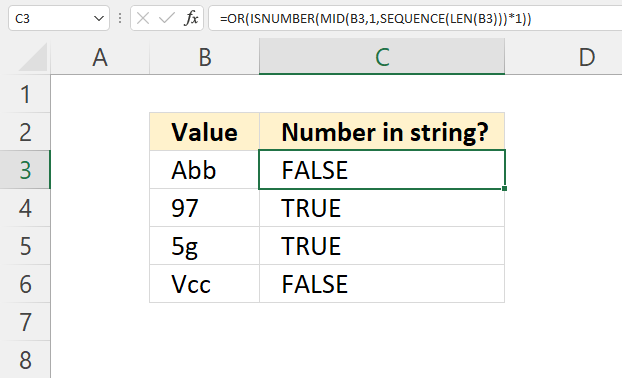The image above shows a formula in cell C3 that returns TRUE if at least one character in the string is a digit.

Excel 365 formula in cell C3:

=OR(ISNUMBER(MID(B3, 1, SEQUENCE(LEN(B3)))*1))

Alternative array formula:

=OR(ISNUMBER(SEARCH({1; 2; 3; 4; 5; 6; 7; 8; 9},B3)))

### 8.1 Explaining formula in cell C3

#### Step 1 - Count characters

The LEN function returns the number of characters in a given string.

LEN(value)

LEN(B3)

becomes

LEN("Abb")

and returns 3.

#### Step 2 - Create a sequence of numbers from 1 to n

The SEQUENCE function creates a list of sequential numbers

SEQUENCE(rows, [columns], [start], [step])

SEQUENCE(LEN(B3))

becomes

SEQUENCE(3)

and returns {1; 2; 3}.

#### Step 3 - Split characters in string one by one

The MID function returns a substring from a string based on the starting position and the number of characters you want to extract.

MID(textstart_numnum_chars)

MID(B3, 1, SEQUENCE(LEN(B3)))

becomes

MID(B3, 1, {1; 2; 3})

becomes

MID("Abb", 1, {1; 2; 3})

and returns {"A"; "b"; "b"}.

#### Step 4 - Multiply characters by 1

The asterisk character lets you multiply values in an Excel formula. This step is needed in order to convert digits to numbers. For example, "2" becomes 2.

ISNUMBER("2") returns FALSE and we don't want that.

MID(B3, 1, SEQUENCE(LEN(B3)))*1

becomes

{"A"; "b"; "b"}*1

and returns

{#VALUE!; #VALUE!; #VALUE!}. The error value appears when we try to multiply a letter with a number. It is simply not possible.

#### Step 5 - Check if charcater is a number

ISNUMBER(MID(B3, 1, SEQUENCE(LEN(B3)))*1)

becomes

ISNUMBER({#VALUE!; #VALUE!; #VALUE!})

and returns

{FALSE; FALSE; FALSE}.

#### Step 6 - Check if at least one character is a digit

The OR function returns TRUE if at least one of the values is TRUE.

OR(ISNUMBER(MID(B3, 1, SEQUENCE(LEN(B3)))*1))

becomes

OR({FALSE; FALSE; FALSE})

and returns FALSE. No digit in this string.